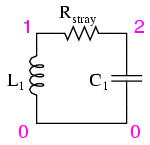# Learning Electronics

Learn to build electronic circuits

# Inductor-capacitor "tank" circuit

PARTS AND MATERIALS

• Oscilloscope
• Assortment of non-polarized capacitors (0.1 µF to 10 µF)
• Step-down power transformer (120V / 6 V)
• 10 kΩ resistors
• Six-volt battery

The power transformer is used simply as an inductor, with only one winding connected. The unused winding should be left open. A simple iron core, single-winding inductor (sometimes known as a choke) may also be used, but such inductors are more difficult to obtain than power transformers.

CROSS-REFERENCES

Lessons In Electric Circuits, Volume 2, chapter 6: "Resonance"

LEARNING OBJECTIVES

• How to build a resonant circuit
• Effects of capacitor size on resonant frequency
• How to produce antiresonance

SCHEMATIC DIAGRAMILLUSTRATIONINSTRUCTIONS

If an inductor and a capacitor are connected in parallel with each other, and then briefly energized by connection to a DC voltage source, oscillations will ensue as energy is exchanged from the capacitor to inductor and vice versa. These oscillations may be viewed with an oscilloscope connected in parallel with the inductor/capacitor circuit. Parallel inductor/capacitor circuits are commonly known as tank circuits.

Important note: I recommend against using a PC/sound card as an oscilloscope for this experiment, because very high voltages can be generated by the inductor when the battery is disconnected (inductive "kickback"). These high voltages will surely damage the sound card's input, and perhaps other portions of the computer as well.

A tank circuit's natural frequency, called the resonant frequency, is determined by the size of the inductor and the size of the capacitor, according to the following equation:Many small power transformers have primary (120 volt) winding inductances of approximately 1 H. Use this figure as a rough estimate of inductance for your circuit to calculate expected oscillation frequency.

Ideally, the oscillations produced by a tank circuit continue indefinitely. Realistically, oscillations will decay in amplitude over the course of several cycles due to the resistive and magnetic losses of the inductor. Inductors with a high "Q" rating will, of course, produce longer-lasting oscillations than low-Q inductors.

Try changing capacitor values and noting the effect on oscillation frequency. You might notice changes in the duration of oscillations as well, due to capacitor size. Since you know how to calculate resonant frequency from inductance and capacitance, can you figure out a way to calculate inductor inductance from known values of circuit capacitance (as measured by a capacitance meter) and resonant frequency (as measured by an oscilloscope)?

Resistance may be intentionally added to the circuit -- either in series or parallel -- for the express purpose of dampening oscillations. This effect of resistance dampening tank circuit oscillation is known as antiresonance. It is analogous to the action of a shock absorber in dampening the bouncing of a car after striking a bump in the road.

COMPUTER SIMULATION

Schematic with SPICE node numbers:Rstray is placed in the circuit to dampen oscillations and produce a more realistic simulation. A lower Rstray value causes longer-lived oscillations because less energy is dissipated. Eliminating this resistor from the circuit results in endless oscillation.

Netlist (make a text file containing the following text, verbatim):

```tank circuit with loss
l1 1 0 1 ic=0
rstray 1 2 1000
c1 2 0 0.1u ic=6
.tran 0.1m 20m uic
.plot tran v(1,0)
.end
```